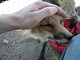View Single Post
#4the_randomizerAlpha Tester Project Supporter Senior Member Join Date: Sep 2008 Location: USA Posts: 1,136Quote:
 Originally Posted by retrobenElementary my dear Watson. Hex numbers 0-F 0 1 2 3 4 5 6 7 8 9 A B C D E F 10 11 12... Decimal numbers 0-9 0 1 2 3 4 5 6 7 8 9 10 11 12 13 14 15 16 17 18... Additionally hex goes A0-FF as well. Hex A0=160 (16 x 10 = 160) Decimal 160=160 Every tens place in hex equals sixteen. Hex 10=16 16=22 1A=26 20=32 (decimals 16+16=32) Decimal 10=10 16=16 20=20 22=22 26=26 32=32 Bits are in the same byte of an address. 8bits=1byte with ten placements. The bits are as follows. bit0? 0=0 neutral bit1 1=1 bit2 2=2 bit3 4=4 bit4 8=8 bit5 10=16 bit6 20=32 bit7 40=64 bit8 80=128 bit9? FF=255 maximum (256th number including zero) It can use any and every mixture of bits. Example:bits 6,5,and 3 20+10+4=34 (52 in decimal). To think,I learned hex all on my own,not in school.
I mean I know 0-255 in hex just fine, it's searching for the right values is the issue when looking at N64 games with the debugger. Then from there, figuring out what does what in the game and so on.
__________________
My rig:
CPU: Intel Core i7 4470 3.4 GHz to 3.9 GHz
Video card:: MSI nVidia GTX 970 4 GB GDDR5
OS: Windows 7 Professional 64-bit
RAM: 16 GB DDR3 SDRAM 10600
HDD: 2 x Western Digital 1 TB HDDs
Monitor: 23" Asus Full HD LED

Oh, and Snes9x > Zsnes in every way